• weixin_43897604 代码整理 function OUT=GrayGradinet(IN) % 灰度梯度共生矩阵 H %归一化灰度梯度矩阵 H_basic %小梯度优势 T1 % 大梯度优势 T2 % 灰度分布的不均匀性 T3 % 梯度分布的不均匀性 T4 % 能量 T5 % 灰度...

代码来自https://tieba.baidu.com/p/586879407?red_tag=0974275182
理论：参考博客1：https://blog.csdn.net/qq_20823641/article/details/51475027
感谢博主 小木匠
理论：参考博客2：
https://blog.csdn.net/weixin_43897604/article/details/102875745
感谢博主 weixin_43897604
原理来自于参考博客2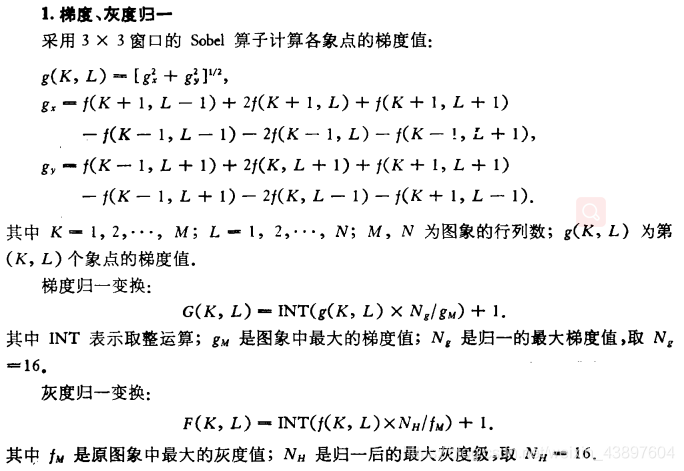代码整理

% 灰度梯度共生矩阵 H
%归一化灰度梯度矩阵 H_basic
%小梯度优势 T1
% 大梯度优势 T2
% 灰度分布的不均匀性 T3
% 梯度分布的不均匀性 T4
% 能量 T5
% 灰度平均 T6
% 梯度平均 T7
% 灰度均方差 T8
% 梯度均方差 T9
% 相关 T10
% 灰度熵 T11
% 梯度熵 T12
% 混合熵 T13
% 惯性 T14
% 逆差矩 T15
gray=256;
% close all;

%  figure, imshow(IN);
gray=256;
[R,C]=size(IN);
%采用平方求和计算梯度矩阵
GM=zeros(R-1,C-1);
for i=1:R-1
for j=1:C-1
n_GM=(IN(i,j+1)-IN(i,j))^2+(IN(i+1,j)-IN(i,j))^2;
GM(i,j)=sqrt(double(n_GM));
end
end
% figure,imshow(GM);
%找出最大值最小值
n_min=min(GM(:));
n_max=max(GM(:));
%把梯度图象灰度级离散化
%设置新的灰度级为new_gray
new_gray=32;
%新的梯度矩阵为new_GM
new_GM=zeros(R-1,C-1);
new_GM=uint8((GM-n_min)/(n_max-n_min)*(new_gray-1));

%计算灰度梯度共生矩阵
%梯度矩阵比轨度矩阵维数少1，忽略灰度矩阵最外围
H=zeros(gray,new_gray);
for i=1:R-1
for j=1:C-1
H(IN(i,j)+1,new_GM(i,j)+1)= H(IN(i,j)+1,new_GM(i,j)+1)+1;
end
end
%归一化灰度梯度矩阵 H_basic
total=i*j;
H_basic=H/total;

%小梯度优势 T1
TT=sum(H);
T1=0;
for j=1:new_gray
T1=T1+TT(1,j)/j^2;
end
T1=T1/total;

%计算大梯度优势 T2
T2=0;
for j=1:new_gray
T2=T2+TT(1,j)*(j-1);
end
T2=T2/total;

%计算灰度分布的不均匀性 T3
T3=0;
TT1=sum(H');
for j=1:gray
T3=T3+TT1(1,j)^2;
end
T3=T3/total;

%计算梯度分布的不均匀性 T4
T4=0;
for j=1:new_gray
T4=T4+TT(1,j)^2;
end
T4=T4/total;

%计算能量 T5
T5=0;
for i=1:gray
for j=1:new_gray
T5=T5+H_basic(i,j)^2;
end
end

%计算灰度平均 T6
TT2=sum((H_basic)');
T6=0;
for j=1:gray
T6=T6+(j-1)*TT2(1,j);
end

%计算梯度平均 T7
T7=0;
TT3=sum(H_basic);
for j=1:new_gray
T7=T7+(j-1)*TT3(1,j);
end

%计算灰度均方差 T8
T8=0;
for j=1:gray
T8=T8+(j-1-T6)^2*TT2(1,j);
end
T8=sqrt(T8);

%计算梯度均方差 T9
T9=0;
for j=1:new_gray
T9=T9+(j-1-T7)^2*TT3(1,j);
end
T9=sqrt(T9);

% 计算相关 T10
T10=0;
for i=1:gray
for j=1:new_gray
T10=T10+(i-1-T6)*(j-1-T7)*H_basic(i,j);
end
end

%计算灰度熵 T11
T11=0;
for j=1:gray
T11=T11+TT2(1,j)*log10(TT2(1,j)+eps);
end
T11=-T11;

%计算梯度熵 T12
T12=0;
for j=1:new_gray
T12=T12+TT3(1,j)*log10(TT3(1,j)+eps);
end
T12=-T12;

%计算混合熵 T13
T13=0;
for i=1:gray
for j=1:new_gray
T13=T13+H_basic(i,j)*log10(H_basic(i,j)+eps);
end
end
T13=-T13;

%计算惯性 T14
T14=0;
for i=1:gray
for j=1:new_gray
T14=T14+(i-j)^2*H_basic(i,j);
end
end

%计算逆差矩 T15
T15=0;
for i=1:gray
for j=1:new_gray
T15=T15+H_basic(i,j)/(1+(i-j)^2);
end
end

x=1:50:750;
OUT(1,1)=T1;
OUT(1,2)=T2;
OUT(1,3)=T3;
OUT(1,4)=T4;
OUT(1,5)=T5;
OUT(1,6)=T6;
OUT(1,7)=T7;
OUT(1,8)=T8;
OUT(1,9)=T9;
OUT(1,10)=T10;
OUT(1,11)=T11;
OUT(1,12)=T12;
OUT(1,13)=T13;
OUT(1,14)=T14;
OUT(1,15)=T15;
% if num>2
%     plot(x,OUT,'-');
%     hold on;
% else
%     plot(x,OUT,'-*r');
%     hold on;
% end

总觉得哪里怪怪的，灰度好像没有离散化，归一化是不是？

展开全文• 概念：像素灰度在空间位置上的反复出现形成图像的纹理，GLCM是描述具有某种空间位置关系两个像素灰度的联合分布。 链接：https://blog.csdn.net/Swithunm/article/details/87932630 在图像中任意一点（x,y）及偏离...

灰度共生矩阵

一个简单的例子

概念：像素灰度在空间位置上的反复出现形成图像的纹理，GLCM是描述具有某种空间位置关系两个像素灰度的联合分布。

链接：https://blog.csdn.net/Swithunm/article/details/87932630

在图像中任意一点（x,y）及偏离它的一点(x+a,y+b)(其中a，b为整数，认为定义)构成点对。设该点对的灰度值为(f1,f2),假设图像的最大灰度级为L,则f1与f2的组合共有L*L种。对于整福图像，统计每一种(f1,f2)值出现的次数，然后排列成一个方阵，再用(f1,f2)出现的总次数将它们归一化为出现的概率P(f1,f2)，由此产生的矩阵为灰度共生矩阵。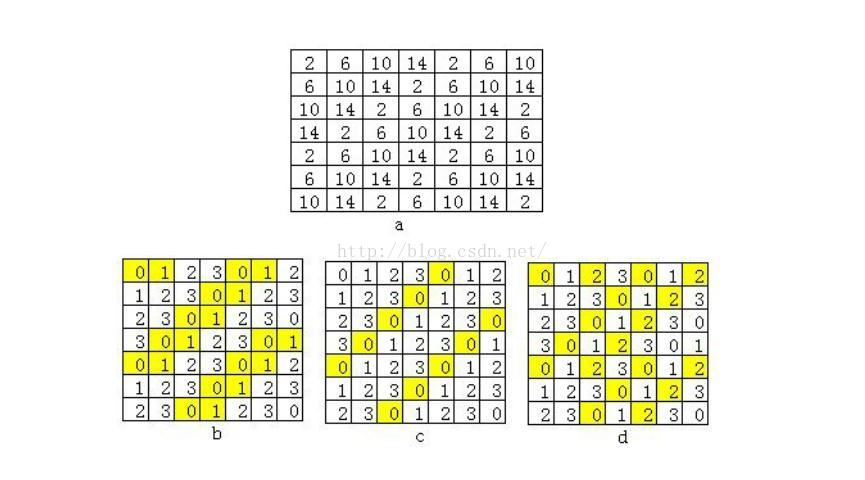灰度共生矩阵特征量

共生矩阵实际上是两个像素点的联合直方图，对于图像中细而规则的纹理，成对像素点的二维直方图倾向于均匀分布；对于粗而规则的纹理，则倾向于最对角分布。

1. 对比度
度量 矩阵的值是如何分布和图像中局部变化的多少，反应了图像的清晰度和纹理的沟纹深浅。纹理的沟纹越深，反差越大，效果越清晰；反之，对比值小，则沟纹浅，效果模糊。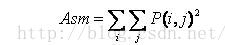2. 能量
能量变换反映了图像灰度分布均匀程度和纹理粗细度。若灰度共生矩阵的元素值相近，则能量较小，表示纹理细致；若其中一些值大，而其它值小，则能量值较大。能量值大表明一种较均一和规则变化的纹理模式。3. 图像包含信息量的随机性度量。当共生矩阵中所有值均相等或者像素值表现出最大的随机性时，熵最大；因此熵值表明了图像灰度分布的复杂程度，熵值越大，图像越复杂。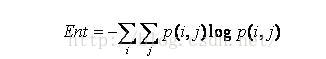4. 逆方差
逆方差反映了图像纹理局部变化的大小，若图像纹理的不同区域间较均匀，变化缓慢，逆方差会较，反之较小。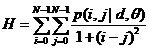5. 相关性
用来度量图像的灰度级在行或列方向上的相似程度，因此值得大小反应了局部灰度相关性，值越大，相关性也越大。参考链接：https://blog.csdn.net/guanyuqiu/article/details/53117507

灰度梯度共生矩阵

灰度梯度共生矩阵模型集中反映了图像中两种最基本的要素，即像点的灰度和梯度（或边缘）的相互关系。各像点的灰度是构成一副图像的基础，而梯度是构成图像边缘轮廓的要素，图像的主要信息是由图像的边缘轮廓提供的。

灰度梯度共生矩阵的分类结果比用灰度共生矩阵好，因为灰度共生矩阵仅用的灰度的信息，而灰度梯度共生矩阵把图像的灰度与梯度信息都用了。

灰度一梯度共生矩阵的元素H( i，j ) 定义为在归 一的灰度图象F( m，n ) 和归一的梯度图象G ( m，n ) 中共同具有灰度为 i 和梯度为 j 的总象点数 . 例如 H ( 10，12 ) =20 , 即图象内象点灰度为10 , 梯度为12的总象点数为20. 以图象的总象点数归一后得到概率为p( i，j )。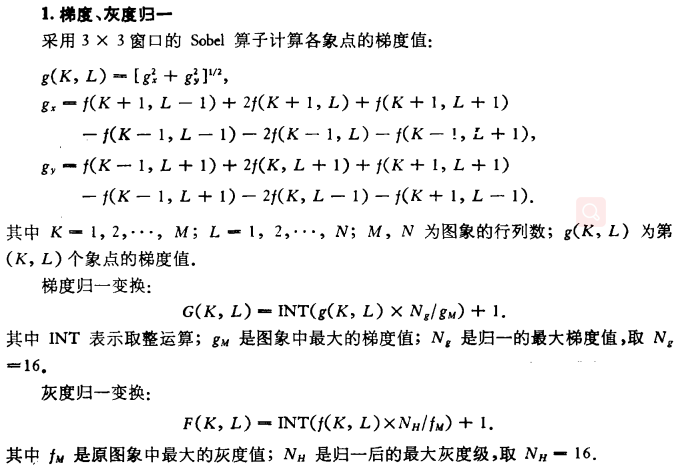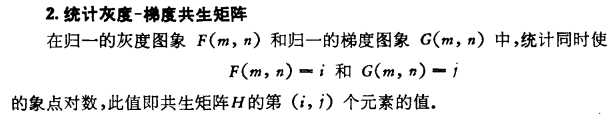摘自论文：洪继光《灰度一梯度共生矩阵纹理分析方法》

展开全文计算机视觉 医学图像处理 灰度共生矩阵 灰度梯度共生矩阵
• 经检验，方便可用，输入为一幅灰度图像，彩色图像可采用rgb2gray转换，输出为图像的15个特征，可广泛应用于图像特征提取，图像检索及图像质量评价领域！
• 我下了一个用灰度梯度共生矩阵提取纹理特征的源程序，现在我处理图像 比如说matlab自带的一幅图像cions.png下面是源程序：function OUT=GrayGradinet(IN)% 灰度梯度共生矩阵 H%归一化灰度梯度矩阵 H_basic%小梯度...

我下了一个用灰度梯度共生矩阵提取纹理特征的源程序，现在我处理图像 比如说matlab自带的一幅图像cions.png

下面是源程序：

% 灰度梯度共生矩阵 H

%归一化灰度梯度矩阵 H_basic

%小梯度优势 T1

% 大梯度优势 T2

% 灰度分布的不均匀性 T3

% 梯度分布的不均匀性 T4

% 能量 T5

% 灰度平均 T6

% 梯度平均 T7

% 灰度均方差 T8

% 梯度均方差 T9

% 相关 T10

% 灰度熵 T11

% 梯度熵 T12

% 混合熵 T13

% 惯性 T14

% 逆差矩 T15

gray=256;

% close all;

%  figure, imshow(IN);

gray=256;

[R,C]=size(IN);

%采用平方求和计算梯度矩阵

GM=zeros(R-1,C-1);

for i=1:R-1

for j=1:C-1

n_GM=(IN(i,j+1)-IN(i,j))^2+(IN(i+1,j)-IN(i,j))^2;

GM(i,j)=sqrt(double(n_GM));

end

end

% figure,imshow(GM);

%找出最大值最小值

n_min=min(GM(:));

n_max=max(GM(:));

%把梯度图象灰度级离散化

%设置新的灰度级为new_gray

new_gray=32;

%新的梯度矩阵为new_GM

new_GM=zeros(R-1,C-1);

new_GM=uint8((GM-n_min)/(n_max-n_min)*(new_gray-1));

%计算灰度梯度共生矩阵

%梯度矩阵比轨度矩阵维数少1，忽略灰度矩阵最外围

H=zeros(gray,new_gray);

for i=1:R-1

for j=1:C-1

H(IN(i,j)+1,new_GM(i,j)+1)= H(IN(i,j)+1,new_GM(i,j)+1)+1;

end

end

%归一化灰度梯度矩阵 H_basic

total=i*j;

H_basic=H/total;

%小梯度优势 T1

TT=sum(H);

T1=0;

for j=1:new_gray

T1=T1+TT(1,j)/j^2;

end

T1=T1/total;

%计算大梯度优势 T2

T2=0;

for j=1:new_gray

T2=T2+TT(1,j)*(j-1);

end

T2=T2/total;

%计算灰度分布的不均匀性 T3

T3=0;

TT1=sum(H');

for j=1:gray

T3=T3+TT1(1,j)^2;

end

T3=T3/total;

%计算梯度分布的不均匀性 T4

T4=0;

for j=1:new_gray

T4=T4+TT(1,j)^2;

end

T4=T4/total;

%计算能量 T5

T5=0;

for i=1:gray

for j=1:new_gray

T5=T5+H_basic(i,j)^2;

end

end

%计算灰度平均 T6

TT2=sum((H_basic)');

T6=0;

for j=1:gray

T6=T6+(j-1)*TT2(1,j);

end

%计算梯度平均 T7

T7=0;

TT3=sum(H_basic);

for j=1:new_gray

T7=T7+(j-1)*TT3(1,j);

end

%计算灰度均方差 T8

T8=0;

for j=1:gray

T8=T8+(j-1-T6)^2*TT2(1,j);

end

T8=sqrt(T8);

%计算梯度均方差 T9

T9=0;

for j=1:new_gray

T9=T9+(j-1-T7)^2*TT3(1,j);

end

T9=sqrt(T9);

% 计算相关 T10

T10=0;

for i=1:gray

for j=1:new_gray

T10=T10+(i-1-T6)*(j-1-T7)*H_basic(i,j);

end

end

%计算灰度熵 T11

T11=0;

for j=1:gray

T11=T11+TT2(1,j)*log10(TT2(1,j)+eps);

end

T11=-T11;

%计算梯度熵 T12

T12=0;

for j=1:new_gray

T12=T12+TT3(1,j)*log10(TT3(1,j)+eps);

end

T12=-T12;

%计算混合熵 T13

T13=0;

for i=1:gray

for j=1:new_gray

T13=T13+H_basic(i,j)*log10(H_basic(i,j)+eps);

end

end

T13=-T13;

%计算惯性 T14

T14=0;

for i=1:gray

for j=1:new_gray

T14=T14+(i-j)^2*H_basic(i,j);

end

end

%计算逆差矩 T15

T15=0;

for i=1:gray

for j=1:new_gray

T15=T15+H_basic(i,j)/(1+(i-j)^2);

end

end

x=1:50:750;

OUT(1,1)=T1;

OUT(1,2)=T2;

OUT(1,3)=T3;

OUT(1,4)=T4;

OUT(1,5)=T5;

OUT(1,6)=T6;

OUT(1,7)=T7;

OUT(1,8)=T8;

OUT(1,9)=T9;

OUT(1,10)=T10;

OUT(1,11)=T11;

OUT(1,12)=T12;

OUT(1,13)=T13;

OUT(1,14)=T14;

OUT(1,15)=T15;

% if num>2

%     plot(x,OUT,'-');

%     hold on;

% else

%     plot(x,OUT,'-*r');

%     hold on;

% end

OUT =

1.0e+004 *

Columns 1 through 8

0.0000    0.0006    0.1618    1.5942    0.0000    0.0103    0.0006    0.0056

Columns 9 through 15

0.0010    0.0196    0.0002    0.0001    0.0003    1.2302    0.0000

下面怎么做呢 ？？？(图像变成什么样子了？，提取出来的共生矩阵是什么)？

[本帖最后由 mooni 于 2009-6-1 13:32 编辑]

展开全文• 提出一种基于Gabor变换和灰度梯度共生矩阵的人耳身份识别方法。首先，利用Gabor变换和灰度-梯度共生矩阵融合提取人耳图像的纹理特征，然后采用K-NN分类器对特征进行分类。该方法用USTB人耳图像库做测试。实验结果...
• 灰度梯度共生矩阵--python

千次阅读 多人点赞 2018-06-06 18:38:34

上一篇博客中，笔者利用python实现了基于灰度共生矩阵方法的纹理特征的提取，本文将利用python实现基于灰度梯度共生矩阵的纹理特征的提取。

可以分布通过公式计算上述15个值，下面只贴出部分公式：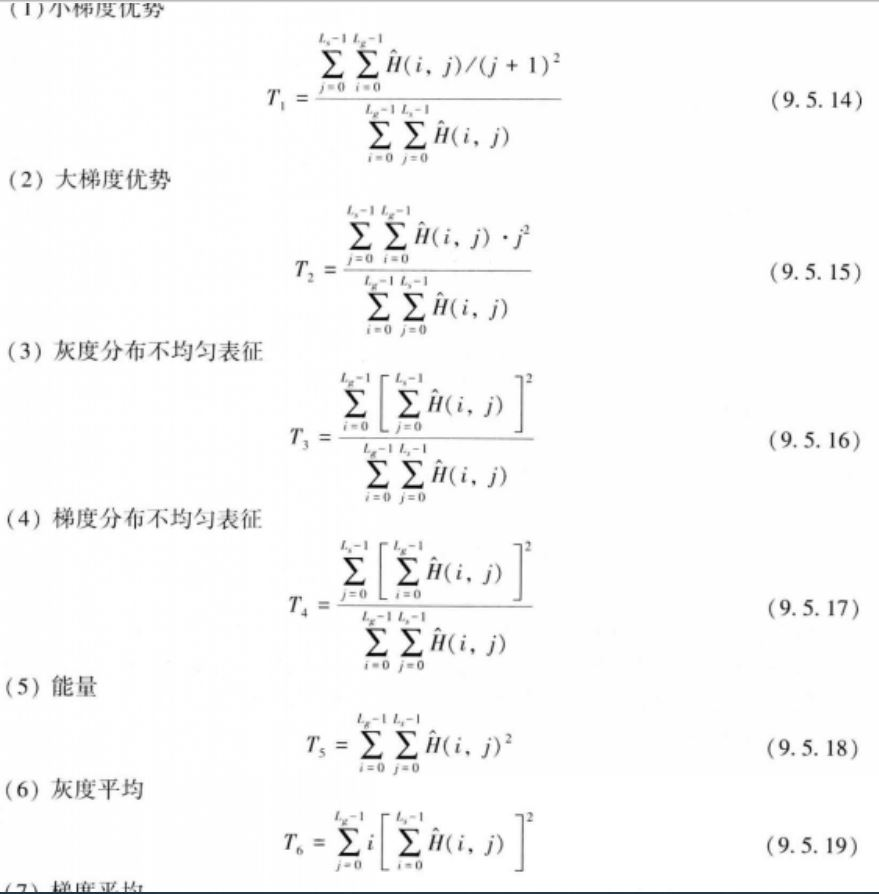本文中利用sobel算子计算图像梯度信息，在得到glgcm矩阵后，计算了上述15个常用的特征值。

import cv2
import numpy as np
from numba import jit
np.set_printoptions(suppress=True) # 输出时禁止科学表示法，直接输出小数值

# 利用sobel算子分别计算x-y方向上的梯度值
gsx = cv2.Sobel(img_gray, cv2.CV_64F, 1, 0, ksize=3)
gsy = cv2.Sobel(img_gray, cv2.CV_64F, 0, 1, ksize=3)
height, width = img_gray.shape
grad = (gsx ** 2 + gsy ** 2) ** 0.5 # 计算梯度值
gray = np.asarray(1.0 * img_gray * (ngray-1) / img_gray.max(), dtype=np.int16) # 0-255变换为0-15
for i in range(height):
for j in range(width):
gray_value = gray[i][j]
return glgcm_features
@jit
def get_glgcm_features(mat):
'''根据灰度梯度共生矩阵计算纹理特征量，包括小梯度优势，大梯度优势，灰度分布不均匀性，梯度分布不均匀性，能量，灰度平均，梯度平均，
灰度方差，梯度方差，相关，灰度熵，梯度熵，混合熵，惯性，逆差矩'''
sum_mat = mat.sum()
gray_variance = grads_variance = corelation = gray_entropy = grads_entropy = entropy = inertia = differ_moment = 0
for i in range(mat.shape):
gray_variance_temp = 0
for j in range(mat.shape):
small_grads_dominance += mat[i][j] / ((j + 1) ** 2)
big_grads_dominance += mat[i][j] * j ** 2
energy += mat[i][j] ** 2
if mat[i].sum() != 0:
gray_entropy -= mat[i][j] * np.log(mat[i].sum())
if mat[:, j].sum() != 0:
grads_entropy -= mat[i][j] * np.log(mat[:, j].sum())
if mat[i][j] != 0:
entropy -= mat[i][j] * np.log(mat[i][j])
inertia += (i - j) ** 2 * np.log(mat[i][j])
differ_moment += mat[i][j] / (1 + (i - j) ** 2)
gray_variance_temp += mat[i][j] ** 0.5

gray_asymmetry += mat[i].sum() ** 2
gray_mean += i * mat[i].sum() ** 2
gray_variance += (i - gray_mean) ** 2 * gray_variance_temp
for j in range(mat.shape):
for i in range(mat.shape):
grads_asymmetry += mat[:, j].sum() ** 2
grads_mean += j * mat[:, j].sum() ** 2
gray_asymmetry /= sum_mat
gray_variance = gray_variance ** 0.5
for i in range(mat.shape):
for j in range(mat.shape):
corelation += (i - gray_mean) * (j - grads_mean) * mat[i][j]
return np.round(glgcm_features, 4)

if __name__=='__main__':
fp = '/home/mamq//images/3.jpg'
img_gray = cv2.cvtColor(img, cv2.COLOR_BGR2GRAY)
glgcm_features = glgcm(img_gray, 15, 15)
print glgcm_features

建议可以使用numba的jit装饰器，在数据量较大的时候能够提高效率。

展开全文python 灰度梯度共生矩阵 纹理特征
• 灰度共生矩阵和灰度梯度共生矩阵的提取方式，是比较重要的纹理特征提取方法，用matlab实现的
• 灰度-梯度共生矩阵的元素定义为在归一化的灰度图像和归一化的梯度图像中共同具有灰度为和梯度为的像素点数。例如，表示图像内像素点灰度为10，梯度为5的像素点个数是45。 1.灰度归一化 其中是归一化后的最大灰度...
•图像分类最小二乘支持向量机 纹理特征
• 纹理特征提取技术，包括灰度共生矩阵灰度梯度矩阵，用于图像的分类识别共生矩阵
• 在分析小波域灰度梯度共生矩阵对梯度特性描述的基础上，设计了一种模糊度评价指标．在遥感影像小波域统计特性，即不同子带对噪声和模糊敏感程度不同的基础上，没计了一种抗噪声的遥感影像模糊度评价指标．实验表明：...
• 灰度梯度共生矩阵纹理特征

万次阅读 2016-05-22 14:32:13
灰度梯度共生矩阵 一、灰度梯度共生矩阵的感知  灰度梯度共生矩阵模型集中反映了图像中两种最基本的要素，即像点的灰度和梯度（或边缘）的相互关系。各像点的灰度是构成一副图像的基础，而梯度是构成图像边缘轮廓的...
• 关于灰度-梯度共生矩阵的经典论文，费了好大的劲收集的，拿出来和大家共享
• 以Hu矩组及灰度梯度共生矩阵为特征参数的磨粒自动分类_朱江共生矩阵
• 此程序将一幅图像的灰度梯度共生矩阵及相关参数算出
•灰度共生矩阵
• MATLAB 灰度共生矩阵 纹理特征提取 粗糙度、对比度、方向度等,源代码纹理特征提取
• 我自己实现的灰度共生矩阵提取结肠癌图像特征，并利用计算机辅助诊断的方法SVM分类Matlab代码。
• 灰度-梯度共生矩阵模型的加权条件熵阈值法张弘;范九伦【期刊名称】《计算机工程与应用》【年(卷),期】2010(046)006【摘要】基于二维灰度直方图的最大熵阈值法是依据"灰度-灰度均值"构成直方图的图像分割方法,该方法...
• 通过纹理分析,利用灰度共生矩阵惯性矩特征值能够反映图像灰度空间复杂度的特性,成功获取了LOG边缘检测算子最佳空间系数,抑制了图像中的大部分噪声.并通过基于TMS320C6000专用信号处理器的图像处理系统实现了图像边缘...matlab 矩阵 开发语言
• 金文图像识别的关键步骤是提取金文的图像特征,针对金文的形态特点,提出了一种基于方向梯度直方图(HOG)和灰度共生矩阵(GLCM)的金文图像识别算法。使用双边滤波对金文图像进行预处理,针对金文的结构特征和局部纹理特征...
• 因为OpenCV中自带的灰度共生矩阵的计算使用过程中，经常出现问题；之前在项目中使用了别人基于OpenCV重新编写cl_texture的灰度共生矩阵，但该代码只能在MFC环境下使用，且不能释放内存；现有的网上流行的基于OpenCV...
• 纹理特征提取方法：LBP, 灰度共生矩阵

万次阅读 多人点赞 2017-05-10 17:21:27
纹理特征提取方法：LBP, 灰度共生矩阵在前面的博文《图像纹理特征总体简述》中，笔者总结了图像纹理特征及其分类。在这里笔者对其中两种算法介绍并总结。参考网址： 《纹理特征提取》 《【纹理特征】LBP 》 ...图像处理 纹理特征提取 算法...